# Eigen value

(diff) ← Older revision | Latest revision (diff) | Newer revision → (diff)

of an operator (transformation)of a vector spaceover a fieldAn element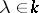such that there is a non-zero vector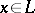satisfying the condition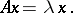This vectoris called an eigen vector ofcorresponding to the eigen value. In the case whenis a linear operator, an eigen value is an elementsuch that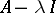is not injective, whereis the identity operator. Ifis a finite-dimensional space, then the eigen values coincide with the roots (in) of the characteristic polynomial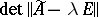, where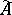is the matrix ofin a certain basis andis the identity matrix. The multiplicity of an eigen value as a root of this polynomial is called its algebraic multiplicity. For any linear transformation of a finite-dimensional space over an algebraically closed field, the set of eigen values is non-empty. Both conditions, finite-dimensionality and being algebraically closed, are essential. For example, a rotation of the Euclidean plane (with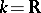) through any angle not divisible byhas no eigen values. On the other hand, for operators on a Hilbert space which are adjoints of (one-sided) shifts, every point of the open unit disc is an eigen value.

The set of all eigen values of a linear transformation of a finite-dimensional space is called the spectrum of the linear transformation. A linear transformation of an-dimensional space is diagonalizable (that is, there is a basis in which the corresponding matrix is diagonal) if and only if the algebraic multiplicity of every eigen value is equal to its geometric multiplicity, which is the dimension of the eigen space (see Eigen vector) corresponding to the given eigen value. In particular, a linear transformation is diagonalizable if it hasdistinct eigen values.

An eigen value of a square matrixover a field(or a characteristic root of) is a root of its characteristic polynomial.

For references see Linear transformation; Matrix.